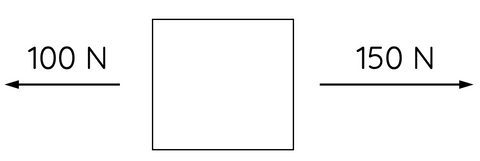# Net Force: A Guide for HSC Physics Students

This topic is part of the HSC Physics course under the section Forces.

### HSC Physics Syllabus

• explore the concept of net force and equilibrium in one-dimensional and simple two-dimensional contexts using: (ACSPH050)

– vector addition by resolution into components

### What is Net Force?

In the HSC Physics course, the concepts of net force and equilibrium are essential for understanding the behaviour of objects in motion or at rest.

### Net Force in HSC Physics

Net force is the vector sum of all individual forces acting on an object. It determines the overall effect of the forces and dictates whether the object will accelerate, decelerate, or remain at a constant velocity. The net force can be calculated using vector addition in both one-dimensional and two-dimensional contexts.

One-dimensional Net Force

In a one-dimensional context, forces act along a straight line. The net force can be calculated by adding the magnitudes of forces acting in the same direction and subtracting those acting in the opposite direction. This concept is essential for understanding linear motion in the HSC Physics course.For example, the net force in diagram above is given by

$$F_{net}=150 - 100$$

$$F_{net}=50 \hspace{1mm} N$$

Therefore, the net force is 50 N to the right.

Two-dimensional Net Force

In a two-dimensional context, forces act in a plane, and their components need to be considered separately along the x and y axes. The net force can be calculated by vector addition, combining the x-components of the forces and the y-components separately to determine the net force in each direction.

Let's consider an example where a mass is acted by multiple forces as shown below.Before we can determine the net force in x and y axes, the 150 N force vector must be resolved into its x and y components.

$$F_{x-component}=150\cos{40º}$$

$$F_{y-component}=150\sin{40º}$$

The net force in the x-direction in the diagram above is given by

$$F_x = 150\cos{40º} - 100$$

$$F_x = 14.9 \hspace{1mm} N$$

Net force in the x-direction is 14.9 N to the right.

The net force in the y-direction is given by

$$F_y = 150\sin{40º} - 80$$

$$F_y = 16.4 \hspace{1mm} N$$

Net force in the y-direction is 16.4 N upwards.

The net force in the x and y direction can be added to find the resultant net force. The magnitude of this resultant net force is given by Pythagorean theorem.

$$F_{resultant}^2=14.9^2 + 16.4^2$$

$$F_{resultant} = \sqrt{14.9^2 + 16.4^2}$$

$$F_{resultant} = 21.1 \hspace{1mm} N$$

The direction of the resultant net force can be determined using trigonometry.

$$\tan{\theta}=\frac{16.4}{14.9}$$

$$\theta = 48º$$

Therefore, the resultant net force is 21.1 N at 48º above the horizontal or x-axis.